# sub-surface scattering with cdrf

## Introduction

In this paper, Kubo, Dobashi and Morishima present a compelling case for using a curvature dependent reflectance function (CRDF) to simulate sub-surface scattering. The basic idea is that smaller objects with higher curvature exhibit more noticeable sub-surface scattering than flatter ones. Using photon mapping with spheres, the authors found a reasonable relationship between the curvature of the sphere (the inverse of the sphere’s radius) and the radiance across the sphere. With the only examples in the paper shown being fairly close to spherical, I wanted to see if I could use their technique, or a variant of it, to get a cheap sub-surface scattering effect on a more complicated mesh. Like, say, the dragon from Stanford’s 3d scanning repository

## The mathematics

First, the authors of the paper attempt to fit their data using the following CDRF:

$$f_r \lparen \theta, \kappa\rparen = \lparen L_i * g\rparen\lparen\theta\rparen$$

Where is the incident light energy and is a gaussian:

$$L_i \lparen\theta\rparen = \max\lparen\cos\lparen\theta\rparen, 0\rparen$$

As can be seen from figure 2(c) in the paper, this is not a particularly good fit though it does match the data qualitatively with a Lambert type shape at low curvature which spreads at higher curvatures.

However, a better quantitative fit is given by (thanks to Cedrick Collomb for help with this):

$$f_r \lparen\theta, \kappa\rparen = {\frac {1+\kappa} 4}{\frac {\cos\lparen \min\lparen {\frac {\lparen 5 + \kappa\rparen\theta} 4}, \pi \rparen \rparen + 1} {1 + \lparen{\frac {\ln\lparen 1 + 1.7\kappa \rparen\theta} {1.45} }\rparen ^{10} } } + 0.075\lparen 1 - \kappa \rparen$$

Once you have this, it’s time to move on to measuring the curvature of your mesh.

## Mesh curvature

There are a few different techniques out there for determining the local curvature of a mesh, but the two main techniques involve either finding an analytical formula or patch that fits the mesh around the vertex you are interested in, or determining the curvature directly from the vertex and normal information. As this was just a prototype, I went with the more performance friendly, and less work-intensive, option of determining the curvature directly from the mesh.

My first attempt was inspired by this paper where the area of each ring of faces around a vertex is compared to the area of those same faces projected onto a unit sphere. This gives a measure of the Gaussian curvature of a mesh which is an intrinsic property of any surface. When applied to a series of test meshes, the results were less than ideal. The sign of the curvature appears correct, but the magnitude depends heavily on the size of the faces, as you would imagine from the definition.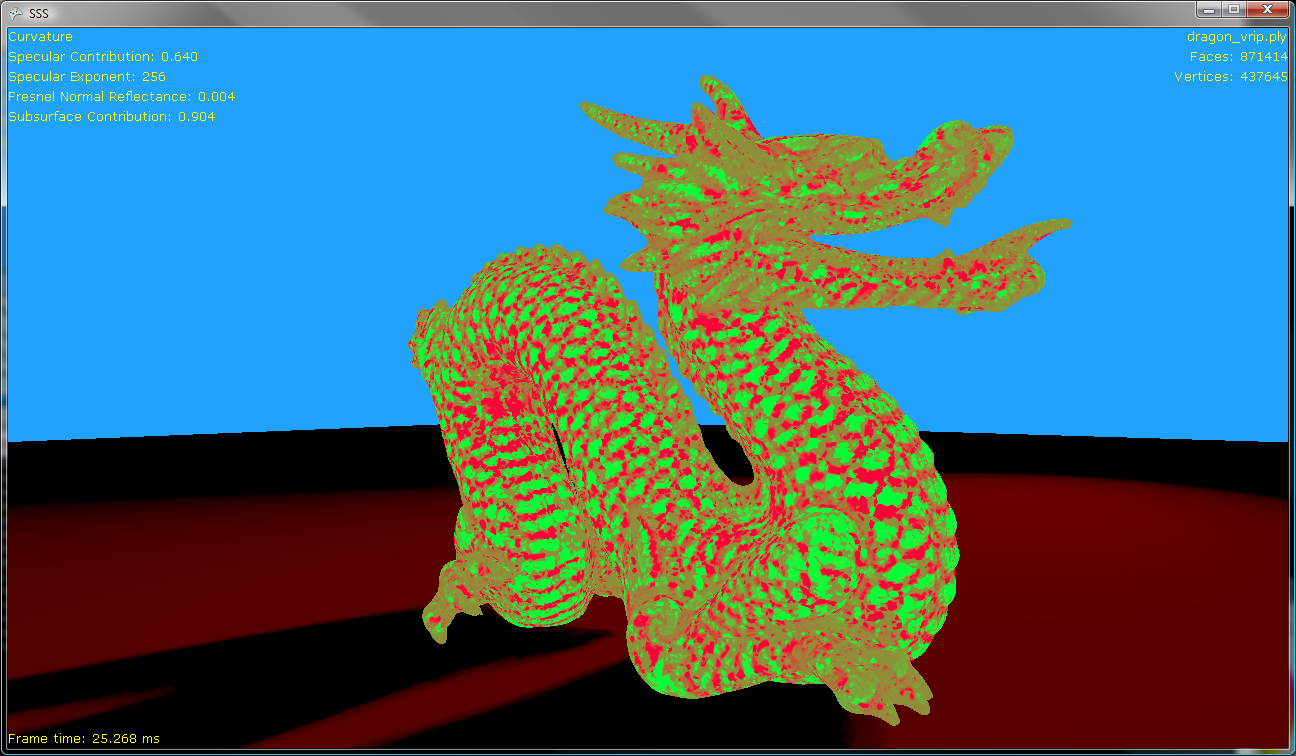I did attempt to extend the neighborhood used to calculate the Gaussian curvature to two rings, but this had two negative effects: The local curvature was smoothed out too much in some places, and other places the technique became invalid as it relies on the sign of curvature not changing within a neighborhood.

These limitations led me to a definition of curvature that is not an intrinsic property of a surface, but depends on the space a surface is embedded in. As I can control the ambient space within my test program and ensure it remains Euclidean (side note – has anyone considered making a game set against a non-Euclidean space?) this measure is still valid for my requirements.

This technique, outlined in this paper again compares areas of faces within the neighborhood of a vertex, but as the area of the face appears in both the numerator and the denominator of the ratio, the magnitudes of curvature are more well-behaved both locally and across the mesh. My results from this technique were far more useful.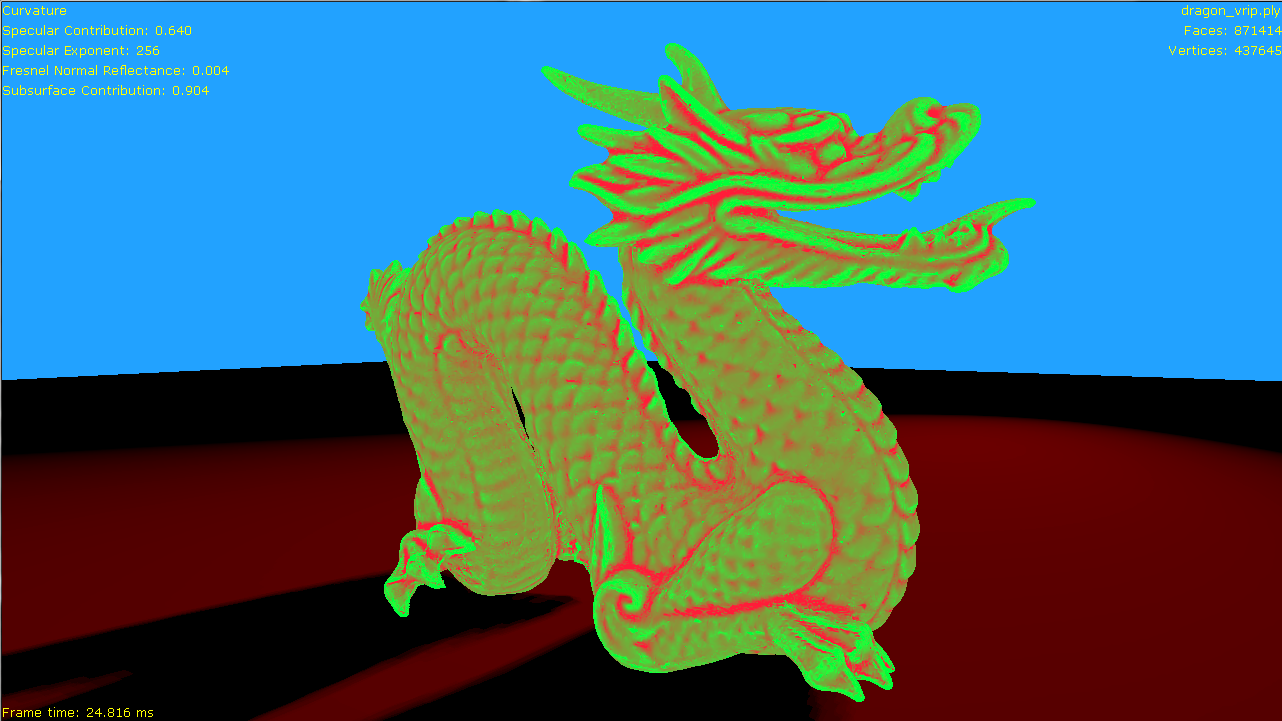## Putting it all together

So now you have an intimidating formula linking local curvature and incident light angle to outgoing radiance. What happens when you put it together?

First, here’s a baseline. The dragon with a simple Lambert BRDF: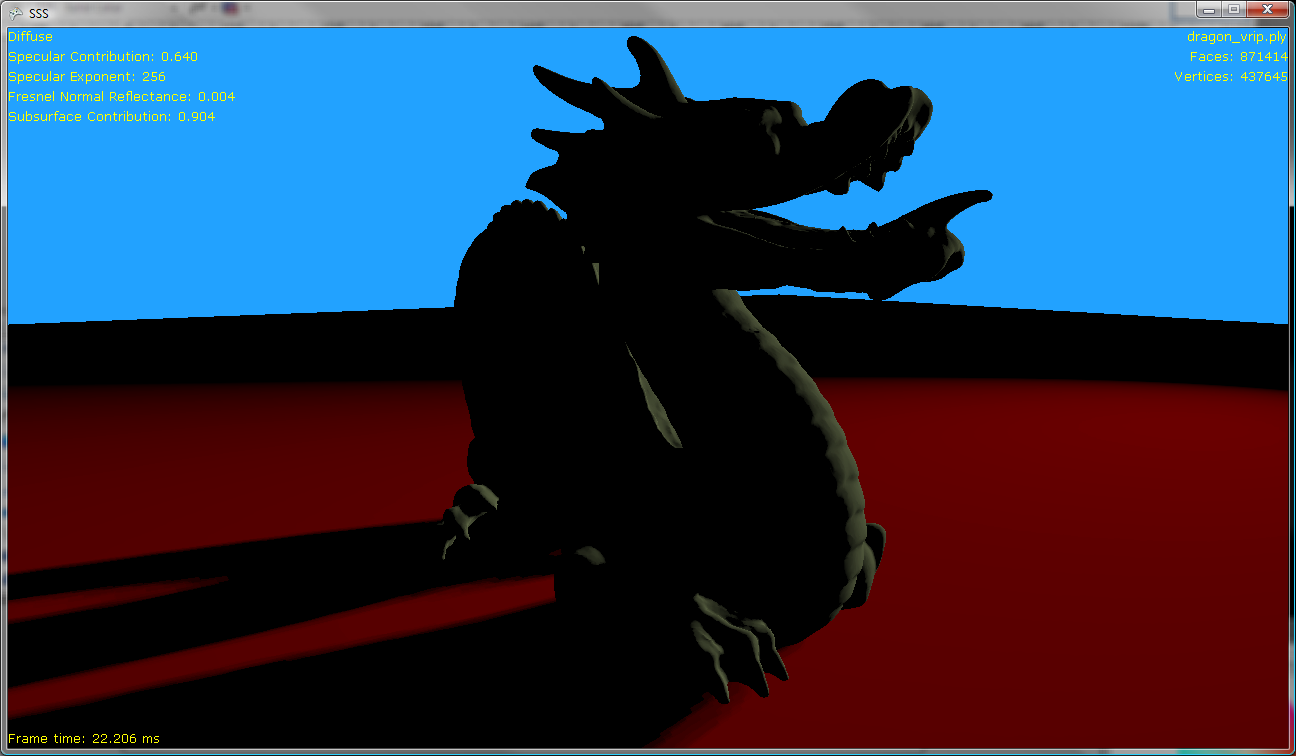Now replacing this with the CDRF from the original paper: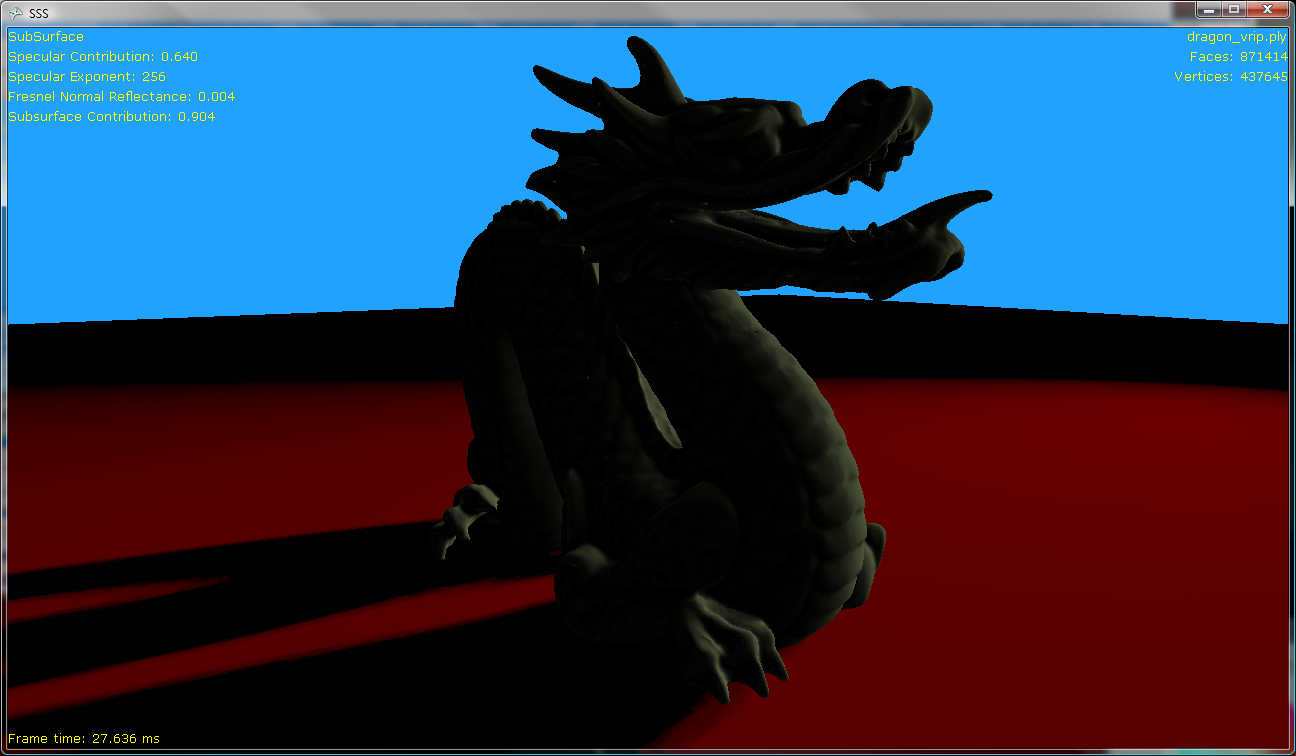The diffuse lighting is clearly wrapping around the model more, as expected, however there are some issues. Note in particular the spine of the dragon which, being thinner, should show more transmission. However, as it has flat curvature when compared to the thicker body of the dragon, it transmits less.

To compare, here’s an image of the same mesh, with the same data, but using a more traditional depth-based technique for sub-surface scattering: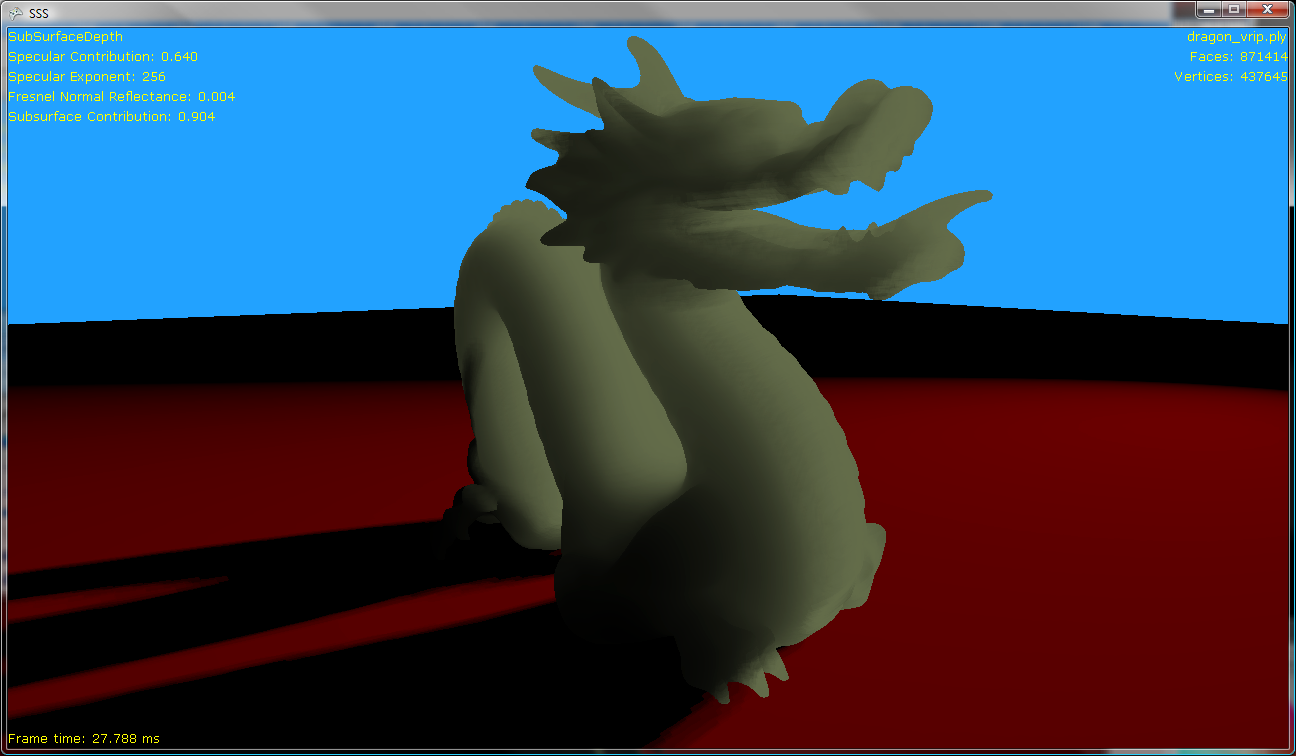Which clearly shows the transmission effect, but where we might expect the scattering effect to be pronounced, around the details in the face, for example, we lose all detail.

While it is true that transmitted light will focus into areas of high curvature, this is an additional effect on top of how far the light has to travel within the model. So perhaps by combining these effects and using the CDRF to add a curvature dependent term to the depth-based sub-surface scattering model, we might get something better than either: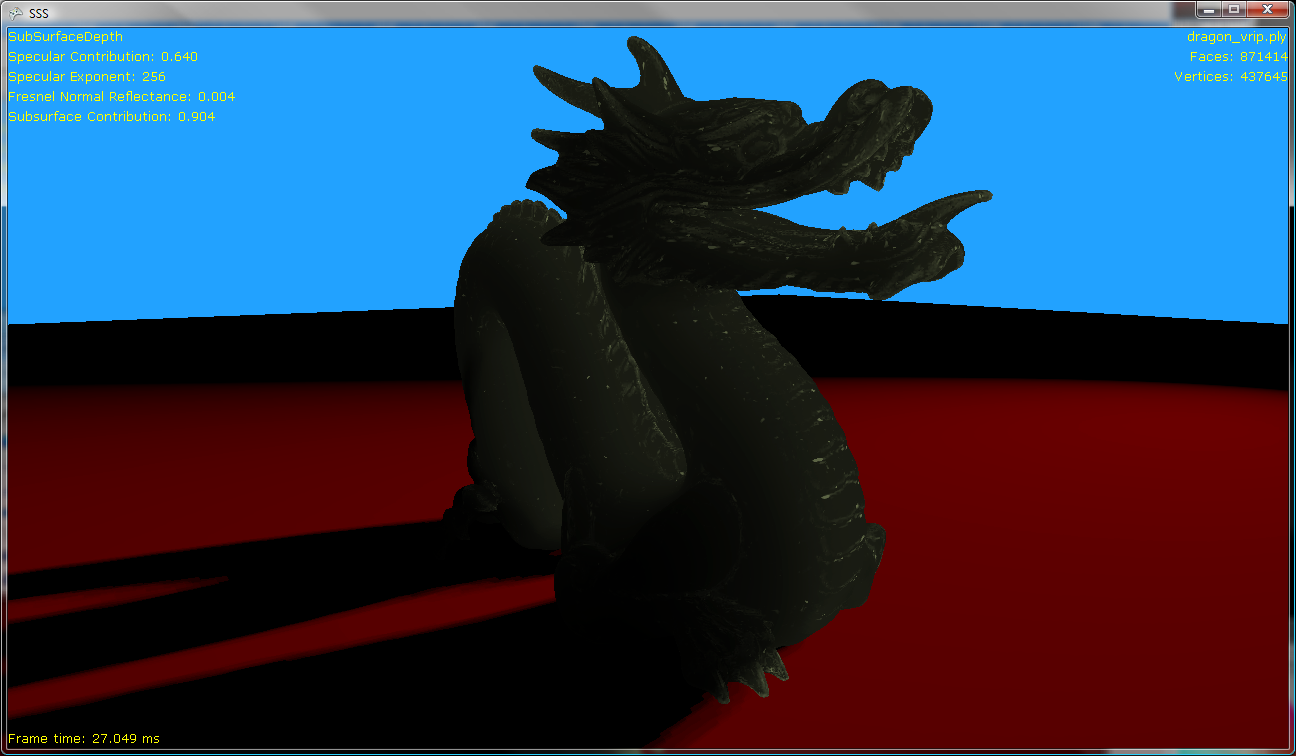Which has all the benefits of a depth-based technique but with the added benefit of concentrating the transmission where the curvature has a high positive magnitude and darkening the transmission where the curvature is negative.

However, we can combine the curvature with the depth-based sub-surface scattering effect in other ways. We can use the curvature of the model directly to lighten and darken the transmitted light. This should add detail back in without the expense of the CDRF calculations and looks like this: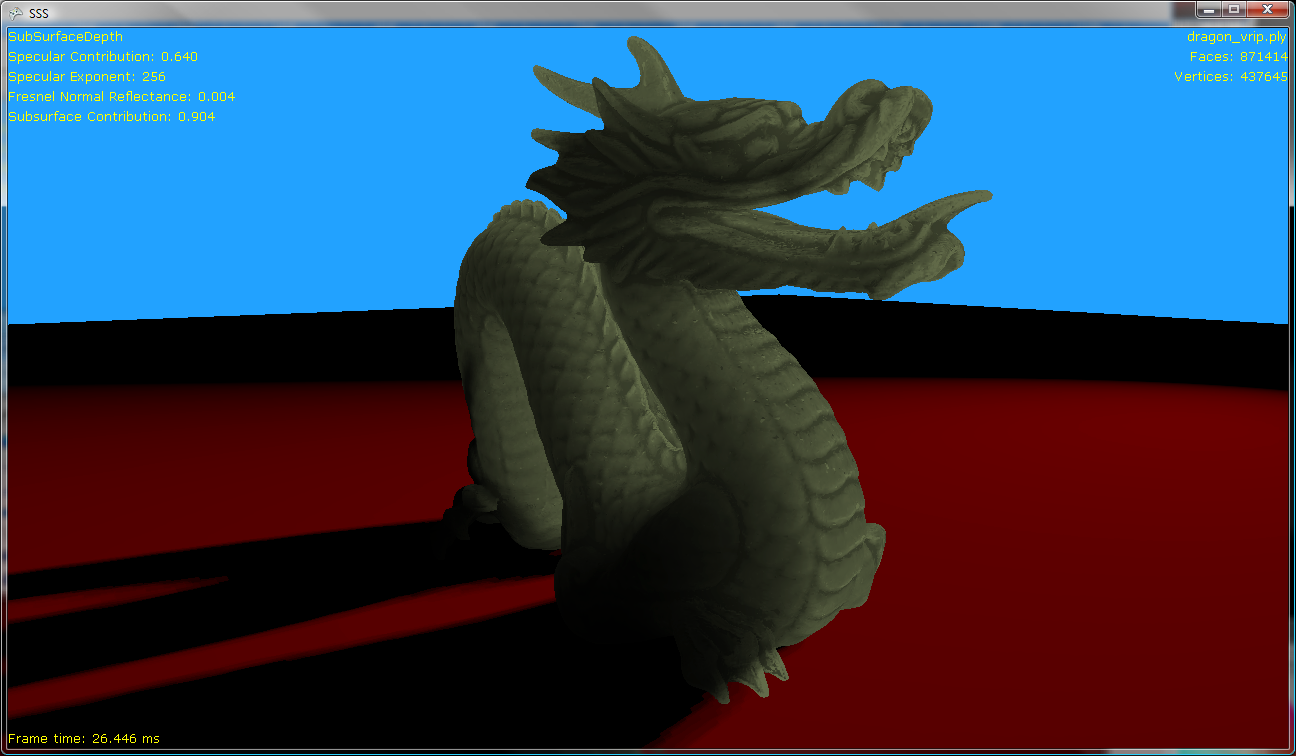This, in combination with a Blinn-Phong BRDF and a Fresnel reflectance term, gives us something that starts to approximate jade: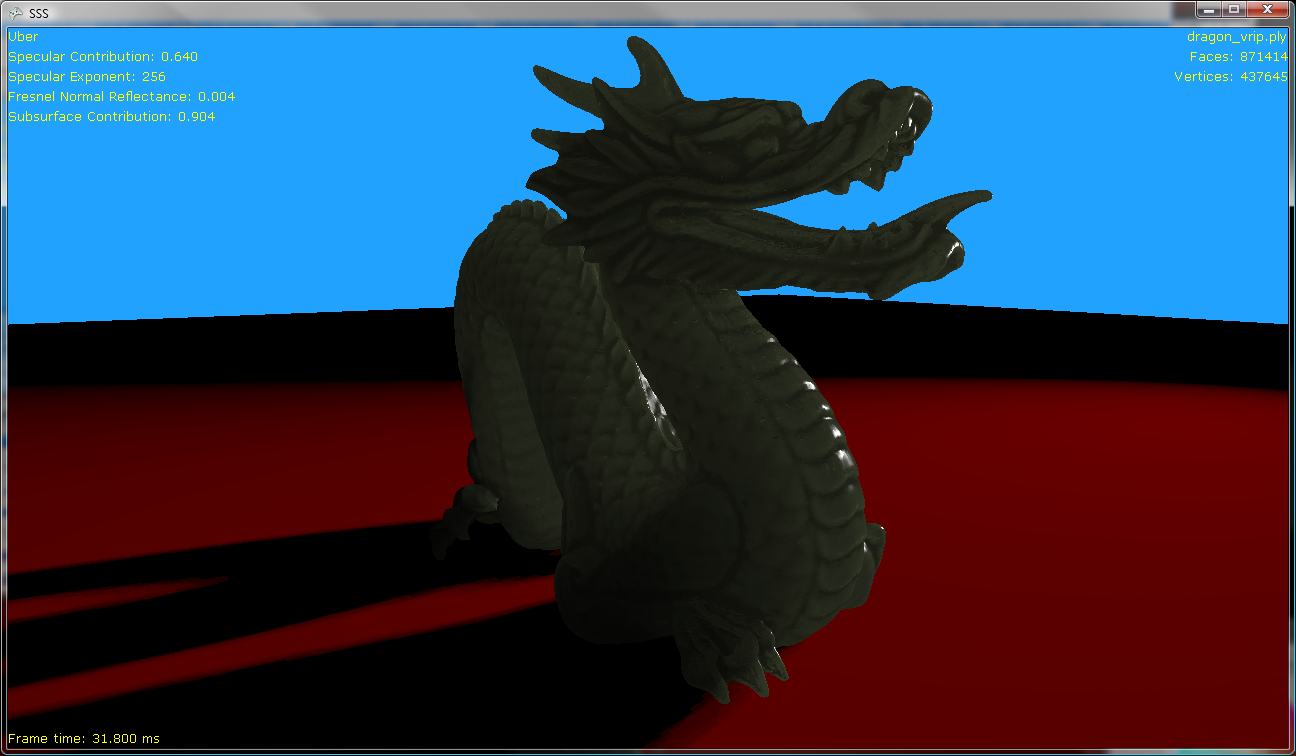## Summary

While the original application of the local curvature of a mesh does not provide a good model of sub-surface scattering for more general cases, it can certainly be applied to existing techniques to add subtlety to the transmission and scattering of light through a model.

these comments are preserved from the original blog post

1. John says:

2010/09/11 at 10:08

hey, nice blog…really like it and added to bookmarks. keep up with good work

2. Kaye Enriguez says:

2010/09/18 at 02:36

What a comment!! Very informative and also easy to understand. Looking for more such writeups!! Do you have a twitter or a facebook?
I recommended it on digg. The only thing that it’s missing is a bit of speed, the pictures are appearing slowly. However thank you for this information.

3. 2010/10/03 at 02:39

[…] This post was mentioned on Twitter by Hirofumi Suda, Hiroyuki KUBO. Hiroyuki KUBO said: おお、どなたか知らんが、追実装してくださっとる。（若干微妙な実装してるきがするが。。。）ありがたや。http://bit.ly/9WIc78 […]

4. Luella Manero says:

Wonderfull, do you happen to have a tweeter i can follow up? really interested.bye

5. Hiroyuki Kubo says:

2010/10/14 at 20:50

Thank you for your interest in my work. I’m an author of that paper.
The model “dragon_vrip.ply” you try to render, it is difficult to compute smooth curvature because it contains artifacts (small spikes) on the surface. I guess it is because of surface reconstruction , but not sure. To compute better, efficient curvature, you had better to use “dragon_vrip_res2.ply”.

Then, I have one question. I cannot understand the equation you post. Where is sigma0?